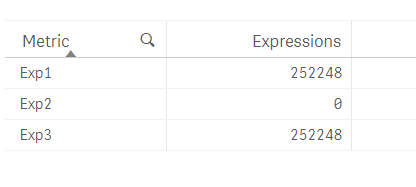# New to Qlik Sense

Discussion board where members can get started with Qlik Sense.

Contributor

## Aggr Expressions NOT Working when using ValueList() to create Synthetic Dimension

I have attached a sample Qlik Sense App that contains a straight table with Custom Dimensions and Expressions.

Here you would see that the second expression DOES NOT generate any output, even though it is the same expression. The only thing that stands out to me is that, the second instance of AGGR in such scenario doesn't work.

Front End TableTable Expression Code

Dimension:

=ValueList('Exp1', 'Exp2', 'Exp3')

Expression:

Pick(Match(ValueList('Exp1', 'Exp2', 'Exp3'), 'Exp1', 'Exp2', 'Exp3')

, Sum(Aggr(Sum(Expression1), Dim1))

, Sum(Aggr(Sum(Expression1), Dim1))

, Sum(Expression1)

)

Any help is appreciated.

1 Solution

Accepted Solutions
Highlighted
Contributor

## Re: Aggr Expressions NOT Working when using Custom Dimension

Here is the solution that worked for me:

Create island tables with the Dummy Dimensions in the Data Model (instead of using ValueList() function to create the synthetic dimensions) and include the Dimension Field in grouping.

Dimension

KPI_Dimension:

Load * INLINE [

KPI_Metric

Exp1

Exp2

Exp3

];

Expression:

Pick(Match(ValueList('Exp1', 'Exp2', 'Exp3'), 'Exp1', 'Exp2', 'Exp3')

, Sum(Aggr(Sum(Expression1), Dim1, KPI_Metric))

, Sum(Aggr(Sum(Expression1), Dim1, KPI_Metric))

, Sum(Expression1)

)

This solves the problem of creating unique aggregations, which is not possible using ValueList(), where the Qlik Engine doesn't understand each dummy value needs a distinct grouping.

3 RepliesMVP

## Re: Aggr Expressions NOT Working when using Custom Dimension

Try

Pick(Match(ValueList('Exp1', 'Exp2', 'Exp3'), 'Exp1', 'Exp2', 'Exp3')

, Sum(TOTAL Aggr( Sum(Expression1), Dim1))

//, Sum(Aggr(Sum(Expression1), Dim1))

, Sum(TOTAL Aggr( Sum(Expression1), Dim1))

, Sum(Expression1)

)MVP

Highlighted
Contributor

## Re: Aggr Expressions NOT Working when using Custom Dimension

Here is the solution that worked for me:

Create island tables with the Dummy Dimensions in the Data Model (instead of using ValueList() function to create the synthetic dimensions) and include the Dimension Field in grouping.

Dimension

KPI_Dimension:

Load * INLINE [

KPI_Metric

Exp1

Exp2

Exp3

];

Expression:

Pick(Match(ValueList('Exp1', 'Exp2', 'Exp3'), 'Exp1', 'Exp2', 'Exp3')

, Sum(Aggr(Sum(Expression1), Dim1, KPI_Metric))

, Sum(Aggr(Sum(Expression1), Dim1, KPI_Metric))

, Sum(Expression1)

)

This solves the problem of creating unique aggregations, which is not possible using ValueList(), where the Qlik Engine doesn't understand each dummy value needs a distinct grouping.